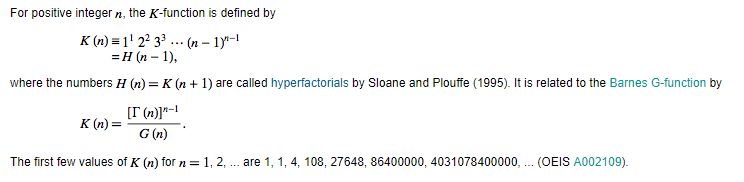# 数学：K函数

In mathematics, the K-function, typically denoted K(z), is a generalization of the hyperfactorial to complex numbers, similar to the generalization of the factorial to the gamma function.

Formally, the K-function is defined asIt can also be given in closed form aswhere ζ'(z) denotes the derivative of the Riemann zeta function, ζ(a,z) denotes the Hurwitz zeta function andAnother expression using polygamma function iswhere A is Glaisher constant.

The K-function is closely related to the gamma function and the Barnes G-function; for natural numbers n, we haveMore prosaically, one may writeThe first values are

1, 4, 108, 27648, 86400000, 4031078400000, 3319766398771200000, … ((sequence A002109 in the OEIS)).